# Tutorial 24: The restrictions on the quantificational rules

Logical System

### Skill to be acquired:

To understand how the various restrictions on the quantificational rules work to exclude certain kinds of invalid inferences

Bergmann The Logic Book Section 10.1

### The Tutorial

#### The Rule of Universal Elimination UE

If a derivation contains a line of the form

n (∀<variable>)<scope> <any justification>

then a line of the form

<<scope>[<constant>/<variable>]> 'n ∀E'

may be added to the derivation. There are no restrictions on this rule.

#### The Rule of Universal Introduction UI

If a derivation contains a line of the form

n. <<scope>[<constant>/<variable>]> <any justification>

then a line of the form

(∀<variable>)<scope> 'n ∀I'

may be added to the derivation, provided that

a) the <constant> does not occur in the <assumptions> of the formula that is generalized (the formula of line n), and
b) the <constant> does not occur in (∀<variable>)<scope>.

If restriction (a) was not there, the rule would permit inferences like Fa ∴ (∀y)Fy thus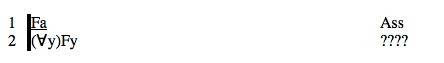(and this inference, perhaps 'Arthur is friendly, therefore Everyone is friendly', is invalid, as we show in the Exercises below).

If restriction (b) was not there, the rule would permit inferences like (∀y)Lyy ∴ (∀x)Lxa thus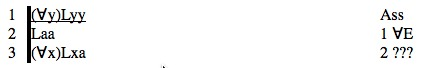(and this inference, perhaps 'Everyone loves him or herself, therefore Everyone loves Arthur', is invalid, also shown below).

#### The Rule of Existential Elimination ∃E

If a derivation contains a line of the form

n. <assumptions> (∃<variable>)(<scope>) <any justification>

and a line of the form

k. <assumptions+ <scope[<constant>/<variable>]>> <formula> <any justification>

and

the constant <constant> does not occur (a) in ∃<variable>)(<scope>) , (b) in any of the <assumptions> of line k except the additional assumption <scope[<constant>/<variable>]>, or (c) in the formula <formula>

then a line of the form

<next line number> <assumptions> <formula> 'k ∃I'

may be added to the derivation.

Roughly what the restrictions are there to prevent is the following. If we have derived an existential formula, say (∃x)Fx, which might be the symbolization of 'Something is F' we can certainly imagine that the principal thing that is F has a name, say 'a', in which case Fa holds ie 'a is F'. But now we have to be careful, because we usually use constants like 'a' to symbolize proper names like 'Arthur' and it certainly does not follow from 'Something is F' that 'Arthur is F'. The naming constant 'a' here has to be, in a manner of speaking, without other properties; it has just to be a temporary bland featureless identification of the thing that is F. If 'a' is already in use in the proof, perhaps Ga is a premise, then the potential exists for mischief for if we infer Fa from (∃x)Fx then we are assuming that the thing which is F is also the one that is G (and this does not follow). The restrictions keep all this in order.

If restriction (a) was not there, the rule would permit inferences like (∃x)Lxx ∴ (∃x)Lxa thusThis inference might be 'Someone loves him or herself, therefore, someone loves Arthur'. It is invalid.

If restriction (b) was not there, the rule would permit inferences like Fa, (∃x)Kx∴ (∃y)(Fy&Ky) thus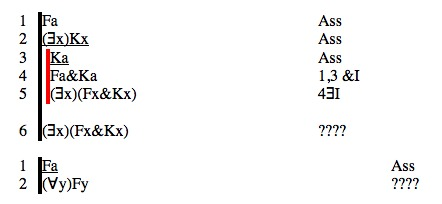This inference might be 'Arthur is friendly, Someone is kind, therefore, Someone is friendly and kind', and it is invalid.

If restriction (c) was not there, the rule would permit inferences like (∃x)Lxa∴ (∃y)Lyy thus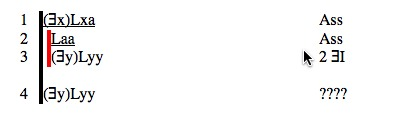This inference might be 'Someone loves Arthur, therefore, someone loves him or her self'. It is invalid.

#### The Rule of Existential Introduction ∃I

If a derivation contains a line of the form

n. <formula>[<constant>/<variable>] <any justification>

then a line of the form

(∃<variable>)<formula> 'n ∃I'

may be added to the derivation. There are no restrictions on this rule.

## Exercise to accompany Predicate Tutorial 14.

The Interpretation in Predex14 shows an Interpretation similar to this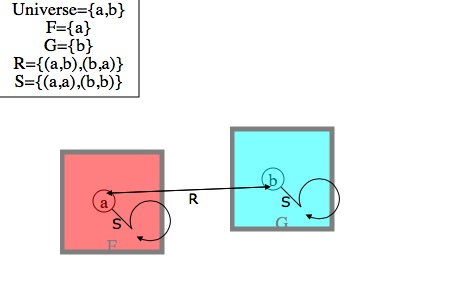if it does not draw a similar one for yourself.

### Exercise 1(of 2)

The rule Universal Introduction has the restriction on it that the constant must not occur in the assumptions of the line on which it is used. The reason for this is that otherwise derivation of some invalid arguments would be possible.

Consider an argument with the constant in the standing assumptions

a) Fa∴ (∀y)Fy

First of all, try to derive it. Then look at it semantically. Is is possible for all the premises to be true and the conclusion false at one and the same time?

Take the interpretation shown in the drawing

Universe= { a,b }
F= { a }

The premise is true, and the conclusion false. It is invalid.

The rule Universal Introduction also has the restriction on it that the constant must not occur in the Universally quantified formula . The reason for this is that otherwise derivation of some invalid arguments would be possible. Consider

b) (∀y)Syy ∴ (∀y)Sya

First try to derive it. The look at it semantically. Is is possible for all the premises to be true and the conclusion false at one and the same time?

Take the interpretation shown in the drawing

Universe= { a,b }
S= { <aa> <bb>}

The premise is true, and the conclusion false. It is invalid.

### Exercise 2 (of 2)

The rule Existential Elimination has on it three restrictions.

First the constant of instantiation must not occur in the proposed target instantiation. Consider the following argument.

(∃x)Lxx ∴ (∃x)Lxa

It is invalid. Try to derive it, then look at the supplied Interpretation.

Second, the constant of instantion must not occur in the assumptions of the step. Consider the following argument.

Fa, (∃x)Kx∴ (∃y)(Fy&Ky)

It is invalid. Try to derive it, then look at the supplied Interpretation.

Third, the constant of instantion must not occur in the existential formula that is instantiated. Consider the following argument.

(∃x)Rxa∴ (∃y)Ryy

It is invalid. Try to derive it, then look at the supplied Interpretation.

Interpretations Widget